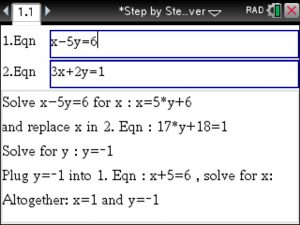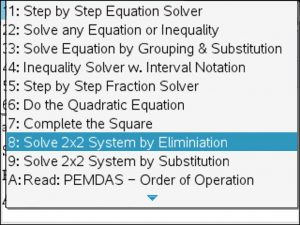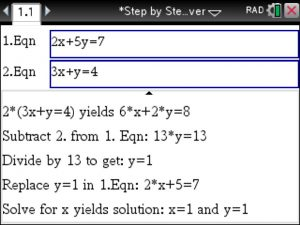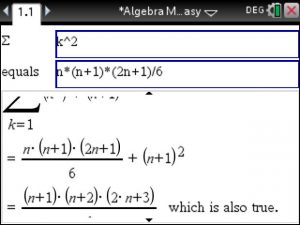## TiNspire Solver with Sign Charts

Solving inequalities using the TiNspire’s Step by Step Equation Solver at www.TiNspireApps.com is straightforward. Go to menu option 5 1:

Next , enter the inequality given :

Scroll down to view the Sign Chart :

Voila!!

## Solving Fractions and Quadratics using the TiNSpire – Step by Step

Say your teacher has some fancy fractions to solve for you and you have a volleyball game , play practice and to prepare for the SAT next Saturday. So you take out your TiNspire CX CAS, launch the STEP BY STEP EQUATION SOLVER app from www.TiNspireApps.com and get a quick lesson on how to solve those fractions…which turns out not too difficult after following the provided steps below:

We select option 5 :

1. problem:

to get 5/14 . The trick is to multiply the given fractions by the product of their denominators (bottoms) to get a much easier equation to solve.

Here is another one:

and

It always works!

Quadratic equations can also be solved step by step. Here is one:

and

Even equations containing only variables can be solved (for x):

Posted on Categories algebra, tinspirecx

## Logarithms – Step by Step – using the TiNspire CX CAS

Using the Step by Step Equation Solver at www.TiNspireApps.com we can solve – step by step – equations involving Logarithms such as

log(x-2)+log(2x+1)=7

just type it in the top box and view the step by step solution in the bottom box:

Another example using natural logarithm instead of base 10 :

Say we are asked to expand logarithms, we will then use the Algebra Made Easy app at www.tinspireapps.com , go to menu option EXPAND, enter our condensed log expression in the top box to view the expanded version as shown below :

and

Lastly , we are given an expanded logarithmic expression and we are asked to condense. Well, you know what to do: use the Algebra Made Easy app at www.tinspireapps.com , go to menu option CONDENSE , enter our expanded log expression in the top box to view the condensed version as shown below :

## Logarithms using the TiNspire CX

Say we have to logarithm base 6 of the cube root of 3 , here is how we enter it into the TiNspire CX CAS:

Upon pressing ENTER we will see the pretty format and the answer:

Let’s do another example: Logarithm base 6 of 1296 :

Pressing ENTER yields the solution 4 (since 1296 = 6^4) :

Here is exponential equation involving logarithm:

This is how it is entered:

Conveniently, log base 10 and exponential base 10 function cancel to get

Now we solve this function:

Voila.

Lastly, evaluate

We enter as

to get

The reason for that clean answer is using the ln rules as follows:

and lastly

We have to solve the following logarithmic equation:

Enter as

log((r+15)^2,3) = 4 and lastly

solve( log((r+15)^2,3) = 4 , r)

which solves for r using the TiNspire cx .

By hand: log_3_(r+15)^2 = 4 calls for exponentiating both sides using base 3 which yields : (r+15)^2 = 3^4 = 81
Square rooting both sides : r+15 = plus or minus 9
Thus, r = -6 or r = -24 . Plug each into the original equation to verify the correctness.

Posted on Categories algebraTags ,

## Solve 2×2 System of Equation – Step by Step – using TiNSpire CX

When solving

x-5y =6
3x+2y=1

with the TiNspire CX CAS  you can either use the Substitution or the Elimination Method.

Let us demonstrate how to use the Substitution Method using the STEP BY STEP EQUATION SOLVER first:Now, to use the Elimination Method select option 1,8 :Below watch the Steps  to solve the system of equations using the Elimination Method:It always works! Always get correct solutions. And you get to select YOUR method of choice.

Posted on Categories algebra, steps, tinspirecx

## College Algebra for the TiNspire CX

No matter where you are, which school you’re attending, algebra class is a requirement. But! With our new algebra app for the TiNspire CX, it will by far your easiest class yet! Not only does this app cover all the material you will learn in class, we provide you with step by step solutions that will significantly boost your understanding of the subject. Don’t believe it? Try our free trial version at www.tinspireapps.com You can check out our college algebra video below for more! Trust our customers—this is the way to go!

Posted on Categories algebra, calculator, tinspirecx

## Math App for the TiNspire CX

It’s here! Our algebra app covers both algebra 1 and algebra 2. You will be acing those tests in no time! Want to check it out? Watch our sneak peek video below, which covers all the jaw-dropping aspects of this extraordinary app. Go to www.tinspireapps.com to try our free trial. Remember, with this app, you’ll understand everything from matrices and polynomials to solving triangles by using the law of sine and the law of cosine. There’s no better way to learn!

Posted on Categories algebra, calculator, tinspirecx

## Texas Instrument Calculator

This app for the TiNspire CX allows you to follow step by steps computations online. You can learn everything from solving polynomials and matrices to the angles and sides of a triangle. Check out our video below for a sneak peek of just what our app can do. Want more? Go to www.tinspireapps.com to test the free trial. The fun continues there!

Posted on Categories algebra, calculator, tinspirecx

## Equation App for the TiNspire CX

Taking Precalculus, Algebra 1 or Algebra 2 and need help with equations? Try our equation app! With no other app will you learn step by step how to solve and simplify equations in any math class you participate in! Take a look at our equation app video below and try our free trial of this equation app at www.tinspireapps.com

Posted on Categories algebra, preCalculus, steps, tinspirecx

## ▷Proof by Induction using the TiNspire CX – Step by Step

Here is how to do Proof by Induction using the TiNspire CX CAS calculator – step by step.

You first launch Algebra Made Easy and select Proof by Induction for Sums in the menu.

Then enter the sigma expression (thats the general formula of the terms to be added) in the top box and the sum-formula in the bottom box as shown below:In Step1 (the base case) we plug in 1 into both sides of the equation. If we get a matching answer we move on to Step 2.Now we assume that the given formula is correct for the n-th integer S(n) (really for the first n integers) and add the n+1. term to both sides of the  equation, simplify the right side and get the expected expression for S(n+1)then proof by induction allowed is to verify the given identity. Voila!!Posted on Categories algebra, tinspirecx